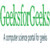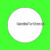# Wand thumbnail() function – Python

• Last Updated : 19 May, 2021

The thumbnail() function is an inbuilt function in the Python Wand ImageMagick library which is used to make the thumbnail image of the given image.

Syntax:

`thumbnail(width, height)`

Parameters: This function accepts four parameters as mentioned above and defined below:

• width: This parameter stores the width of the thumbnail image.
• height: This parameter stores the height of the thumbnail image.

Return Value: This function returns the Wand ImageMagick object.

Original Image:Example 1:

## Python3

 `# Import library from Image``from` `wand.image ``import` `Image` `# Import the image``with Image(filename ``=``'../geeksforgeeks.png'``) as image:``    ``# Clone the image in order to process``    ``with image.clone() as thumbnail:``        ``# Invoke thumbnail function with x as 50 and y as 50``        ``thumbnail.thumbnail(``50``, ``50``)``        ``# Save the image``        ``thumbnail.save(filename ``=``'thumbnail1.jpg'``)`

Output:Example 2:

## Python3

 `# Import libraries from the wand ``from` `wand.image ``import` `Image``from` `wand.drawing ``import` `Drawing``from` `wand.color ``import` `Color` `with Drawing() as draw:``    ``# Set Stroke color the circle to black``    ``draw.stroke_color ``=` `Color(``'black'``)``    ``# Set Width of the circle to 2``    ``draw.stroke_width ``=` `1``    ``# Set the fill color to 'White (# FFFFFF)'``    ``draw.fill_color ``=` `Color(``'white'``)` `    ``# Invoke Circle function with center at 50, 50 and radius 25``    ``draw.circle((``200``, ``200``), ``# Center point``                ``(``100``, ``100``)) ``# Perimeter point``    ``# Set the font style``    ``draw.font ``=` `'../Helvetica.ttf'``    ``# Set the font size``    ``draw.font_size ``=` `30``    ` `    ``with Image(width ``=` `400``, height ``=` `400``, background ``=` `Color(``'# 45ff33'``)) as pic:``        ``# Set the text and its location``        ``draw.text(``int``(pic.width ``/` `3``), ``int``(pic.height ``/` `2``), ``'GeeksForGeeks !'``)``        ``# Draw the picture``        ``draw(pic)``        ``# Invoke thumbnail function with x as 50 and y as 50``        ``pic.thumbnail(``50``, ``50``)``        ``# Save the image``        ``pic.save(filename ``=``'thumbnail2.jpg'``)`

Output:My Personal Notes arrow_drop_up